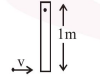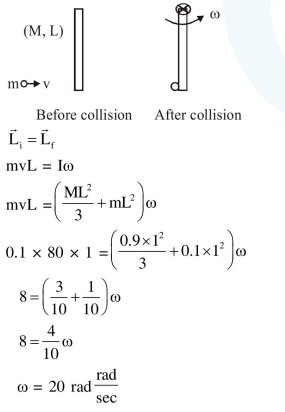# A thin rod of mass

Question:

A thin rod of mass $0.9 \mathrm{~kg}$ and length $1 \mathrm{~m}$ is suspended, at rest, from one end so that it can freely oscillate in the vertical plane. A particle of move $0.1 \mathrm{~kg}$ moving in a straight line with velocity $80 \mathrm{~m} / \mathrm{s}$ hits the rod at its bottom most point and sticks to it (see figure). The angular speed (in $\mathrm{rad} / \mathrm{s}$ ) of the rod immediately after the collision will be________.Solution: